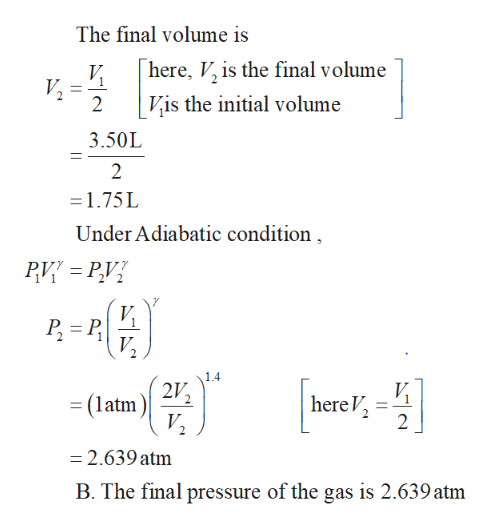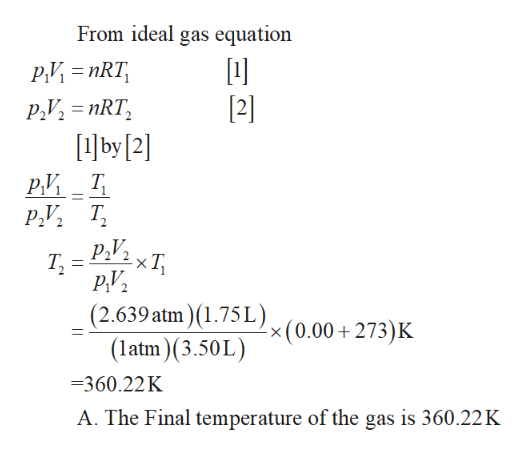# In a cylinder sealed with a piston, you rapidly compress 3.50 L of N2 gas initially at 1.00 atmpressure and 0.00∘C to half its original volume. Assume the N2 behaves like an ideal gas.A)Calculate the final temperature of the gas. Tfinal = ______ ˚C B) Calculate the final pressure of the gas. Pfinal = _______ atm C) If you now cool the gas back to 0.00∘C without changing the pressure, what is its final volume? V final = ________ L

Question

In a cylinder sealed with a piston, you rapidly compress 3.50 L of N2 gas initially at 1.00 atmpressure and 0.00∘C to half its original volume. Assume the N2 behaves like an ideal gas.

A)Calculate the final temperature of the gas.

Tfinal = ______ ˚C

B) Calculate the final pressure of the gas.

Pfinal = _______ atm

C) If you now cool the gas back to 0.00∘C without changing the pressure, what is its final volume?

V final = ________ L
check_circleExpert Solution
Step 1

Given that the final volume of the gas is half of its original volume.help_outlineImage TranscriptioncloseThe final volume is here, V, is the final volume V 2 Vis the initial volume 3.50L 2 1.75L Under Adiabatic condition V VP 2 1.4 2V (latm here V 2 V2 =2.639 atm B. The final pressure of the gas is 2.639 atm fullscreen
Step 2

Write the ideal gas equatio...help_outlineImage TranscriptioncloseFrom ideal gas equation Р.И - nRT, Р., 3 nRT, by  РИ Т Р., Т.  2 Р., РY, (2.639 atm) (1.75L)x(0.00 + 273)K -xT Т, (atm(3.50L) 360.22K A. The Final temperature of the gas is 360.22 K fullscreen

### Want to see the full answer?

See Solution

#### Want to see this answer and more?

Solutions are written by subject experts who are available 24/7. Questions are typically answered within 1 hour*

See Solution
*Response times may vary by subject and question
Tagged in

### Kinetic Theory of Gases